- JoNova - http://joannenova.com.au -

New Science 15: Modeling outgoing radiation (OLR)

Posted By David Evans On October 25, 2015 @ 3:06 pm In Global Warming | Comments Disabled

OLR — outgoing longwave radiation — is so key, so central to the climate debate that if we had top notch data on the radiation  coming off the planet, we would have solved the effect of extra CO2 a long time ago. That we don’t have a specific satellite  monitoring these changes in detail is like the dog that didn’t bark. Apparently a specialist OLR satellite was to be launched in  2015. More info on the RAVAN Satellite here (was supposed to launch in Sept 2015). (UPDATE: Planned for 2016) h/t siliggy.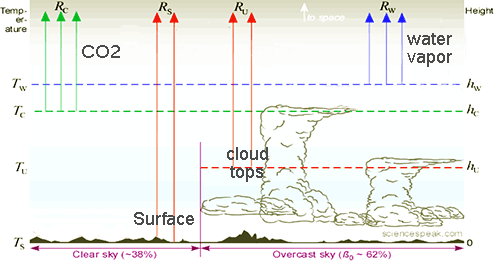There are four main pipes to space, and in David’s work each pipe is considered separately. The conventional model assumes that increasing atmospheric CO2 constricts the CO2 pipe, which warms the surface, causing more evaporation, which then constricts the Water Vapor pipe (this is the “water vapor amplification”, even more constriction of radiation to space by water vapor that forces the surface to emit more by being yet warmer). But the missing hot spot tells us that this theory is wrong. In this OLR model, the water vapor pipe could either expand or constrict. An expansion means a drop in the height of the emissions layer, a descent to a warmer place in the troposphere. The top layer  of any atmospheric “blanket” is where all the action is, and where the photons of infra red can finally escape to space. The gas below the top of the blanket or emission layer doesn’t matter that much for OLR because the photons can’t escape to space from there — there are just too many molecules to capture those rays of infra red before they can get far.

The amount of outgoing radiation emitted by an emission layer always depends entirely on how warm it is, and as the “blanket” of humidity (or water vapor) thickens, the top part of that layer presumably ascends, reaches higher, and becomes cooler, which means it emits less (the pipe constricts). Alternately if the blanket gets thinner near its top (even if getting thicker far below), the height of the emissions layer falls lower into warmer air, so it emits more.

The model estimates how much the radiation to space changes as various properties of the the emission layers and the atmosphere change — the heights of the emission layers, the lapse rate, the surface temperature, the cloud fraction, and the CO2 concentration.

Lapse rate is the rate the temperature falls or lapses per kilometer, as we go up vertically through the atmosphere. We calculate the temperature at a given height by working upwards from the surface, as the surface temperature less the lapse rate (in deg C per km) times the height (in km).

The cloud fraction splits the atmospheric window between the cloud tops and surface emissions.

Incidentally, now that we have the OLR model we can estimate the Planck sensitivity, under those hypothetical Planck conditions.

–Jo

———————————————————-

# 15. The OLR Model

Dr David Evans, 23 October 2015, David Evans’ Basic Climate Models Home, Intro, Previous, Next, Nomenclature.

This post presents a model of outgoing longwave radiation (OLR), which we are soon going to join with the sum-of-warmings model to form the alternative basic climate model, as outlined in the post on modelling strategy.

The last post presented the basic parameters of the various layers that effectively emit OLR. In this post we model the sensitivity of OLR to changes in some of those parameters — for example, how much does OLR decrease if the water vapor emissions layer (WVEL) ascends by 100 meters? See Fig. 1 of that post to see how some of the parameters are defined.

The final results of this series are not very sensitive to the OLR model, which can be refined later if the alternative basic climate model is found to be useful.

If you want skip the details, jump to “The Model Equation” and “Diagram” sections near the end.

### The CO2 Emission Layer

The OLR model needs to know the increase in the OLR emitted by the CO2 emissions layer (CO2EL), ΔRC, when there are increases in the CO2 concentration, the surface temperature, or the lapse rate.

We are going to be holding two of these three factors constant while allowing the other to increase — yes, that is a partial derivative where it is hard to justify holding things constant, but (a) it is difficult to see how to proceed otherwise, and (b) it doesn’t have as much impact on the final outcome as say the Planck sensitivity does in the conventional model. (The alternative model ameliorates the impossible-partial-derivative problem by replacing the Planck sensitivity with the Stefan Boltzmann sensitivity, but the problem is still present in less strategic places such as this.)

For wavelengths around 15 μm the CO2EL is in the stratosphere, whose temperatures are assumed to remain constant when just the surface temperature or lapse rate change (in line with the Planck conditions — see the Nomenclature file above). Thus the “average temperature” of the CO2 emissions layer is of no direct relevance.

#### - CO2 Concentration

When the CO2 concentration doubles and everything else remains constant, RC decreases by(see post 2). The decrease in RC is logarithmic in change of CO2 concentration, so when the CO2 concentration changes from C0 to C0C and all else remains constant,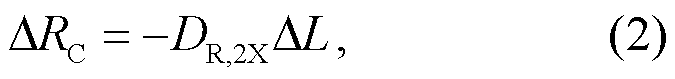where L is the base-2 logarithm of the CO2 concentration C and, as in post 2,The last figure in this page of Barrett-Bellamy illustrates the effect on the OLR spectrum of doubling CO2, which is to slightly widen the indentation centered on 15 μm, with the changes nearly all occurring in the wings.

#### - Surface Temperature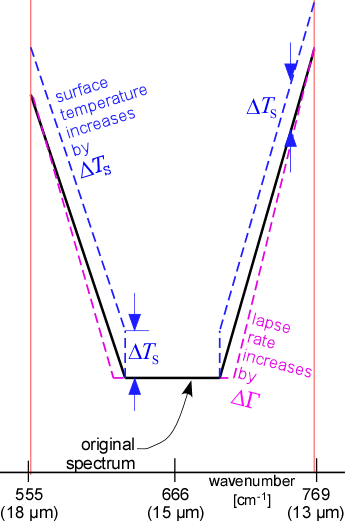Figure 1: Schematic of the indentation in the Earth’s emission spectrum around 15 μm due to CO2. The area under the indentation is approximately proportional to RC, the OLR emitted by the CO2 emission layer. Note the flat bottom of the “well” around 15 μm, at which wavelengths the emission layer is in the stratosphere, and the sloping “wings” on the sides of the well, at which wavelengths the emission layer is in the troposphere (because it takes more CO2 to absorb a photon on average).

The Nimbus emission spectrum in Fig. 2 of the last post is the basis for graphically converting changes in surface temperature TS or lapse rate Γ to changes in RC, following the stylized representation of the indentation in Fig. 1.

If the surface warms by ΔTS and Γ does not change then all the tropospheric air warms by ΔTS, so at each wavelength at which the CO2EL is in the troposphere the CO2EL warms by ΔTS, while in the stratosphere the CO2EL remains unchanged. If ΔTS is 10°C then the area under the indentation increases by ~9.4%, which by Table 1 of the last post corresponds to ~4.6 W m−2. Thus#### - Lapse Rate

If the lapse rate Γ increases while TS remains unchanged, the atmosphere becomes linearly colder as height increases (Γ is positive, ~6.5 °C km−1).

Conventionally it is assumed that the average lapse rate decreases uniformly throughout the troposphere due to surface warming: increased evaporation is assumed to increase the specific humidity throughout the troposphere. But as reported by Paltridge, Arking, and Pook in 2009  from a study of the better radiosonde data from 1973, the extra water vapor is apparently confined to the lower troposphere, and the consequent greater stability at these low altitudes leads to less overturning and less transport of water vapor to the upper troposphere. Humidity data from the radiosondes indicates that since 1973 specific humidity has generally been increasing up to ~550 hPa (about 5 km) , but decreasing at heights above that. So in this OLR model we will consider either a “uniform” lapse rate change, or a “partial” lapse rate where the lapse rate only changes below 5 km.

If Γ increases uniformly by 10% then the area under the indentation decreases by ~3.4%, or, by Table 1 of the last post, ~1.6 W m−2, soBut if Γ increases partially by 10% then the area under the indentation decreases by only ~2.5%, or ~1.2 W m−2, so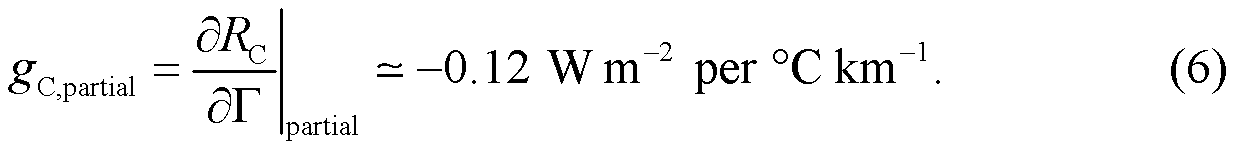#### - Total

The total increase in the OLR from the CO2EL is modeled as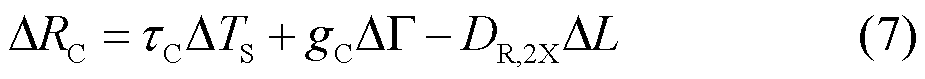where gC is either gC,uniform or gC,partial.

### The Stefan-Boltzmann Emission Layers

For an emission layer whose various emitting parts in the atmosphere are mostly at temperatures relatively close to the mean temperature of the emission layer, the emission spectrum approximately follows a blackbody radiance line described by Planck’s law, such as the dashed lines in Fig. 2 of the previous post (Nimbus spectrum). Such a line, integrated over all wavelengths and directions, gives the Stefan-Boltzmann law. Such an emission layer consists of one or more segments of such a line, where the segments are defined by approximately-constant wavelength limits. The OLR emitted by the layer is thus, approximately, a constant fraction φ of the emissions given by the Stefan-Boltzmann law for the layer’s temperature (φ is between 0 and 1).

The surface, cloud tops, water vapor emissions layer (WVEL), and methane emission layer behave thus. Once the temperature change of such a layer is determined from the changes in the relevant inputs (the surface temperature, the lapse rate, and the height of the emission layer), the approximate relative increase in OLR in response to that warming may be determined by the Stefan-Boltzmann law.

Consider emission layer i, where i is either S (surface), U (cloud), W (water vapor), or M (methane). Its average temperature is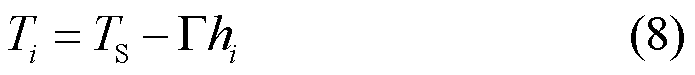where TS is the surface temperature and hi is the average height of the layer (the surface height, hS, is taken as zero). Layer i warms by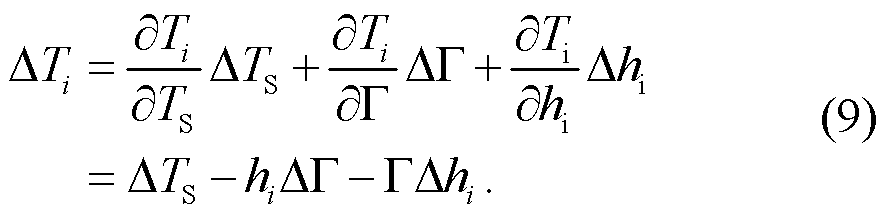Layer i emits OLR Ri of φiσεTi4, the appropriate fraction φi of an all-wavelength Stefan-Boltzmann emitter. Hence the increase in OLR from that layer is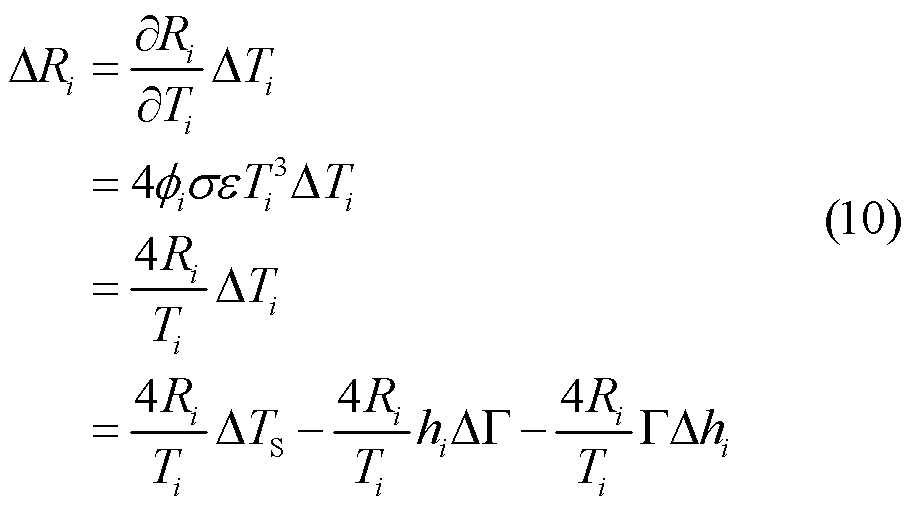where we substituted in Eq. (9) in the last line. Let us define the parameters τi, gi (either gi,uniform or gi,partial, depending on whether ΔΓ applies uniformly or partially) and θi by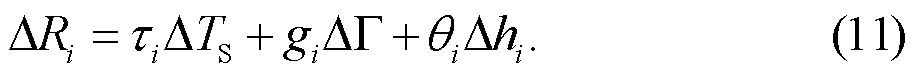Comparing Eq. (10) and Eq. (11) gives the formulae for the values that are calculated in this table (see the spreadsheet for the numeric calculations):

 Emission Layer Subscript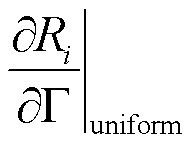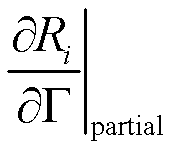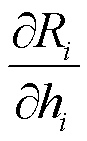Units W m−2 °C−1 W m−2 (°C km−1) −1 W m−2 (°C km−1) −1 W m−2 km−1 Expression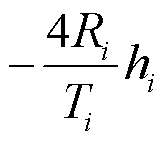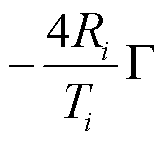Symbol i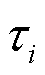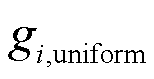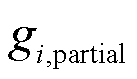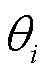Surface S 0.63 0 0 0 Cloud tops U 0.71 −2.4 −2.4 −4.6 Water vapor W 1.34 −10.7 −6.7 −8.7 Methane M 0.08 −0.2 −0.2 −0.5

Table 1: How OLR increases with TS, lapse rate, and height, for the Stefan-Boltzmann emission layers.

### The Ozone Emission Layer

The OLR from the ozone emission layer is assumed constant, because it is above the tropopause and insulated from surface warming, and because ozone levels are assumed constant — or at least unrelated (for now) to changes in TSI, EDA, and CO2. So we take ΔRZ as zero.

### Cloud Fraction and OLR

Under clear skies the OLR in the atmospheric window is ~119 W m−2 from the surface and near surface, while under overcast skies it is ~77 W m−2 from cloud tops. OLR at other wavelengths is not directly affected by clouds. Therefore the increase in OLR due to an increase in the cloud cover fraction β (currently ~62%) is DβΔβ  where Dβ is the difference in emissions between clear and overcast skies: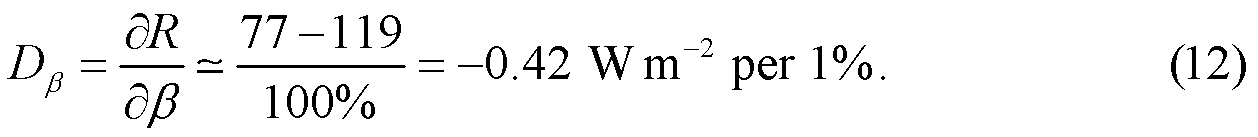### The Model Equation

The total increase in OLR is the sum of its components: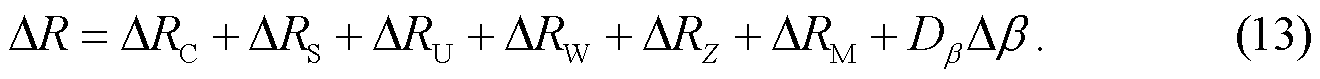Collecting together all the results in the equations above gives us the OLR model as a single equation: the increase in OLR is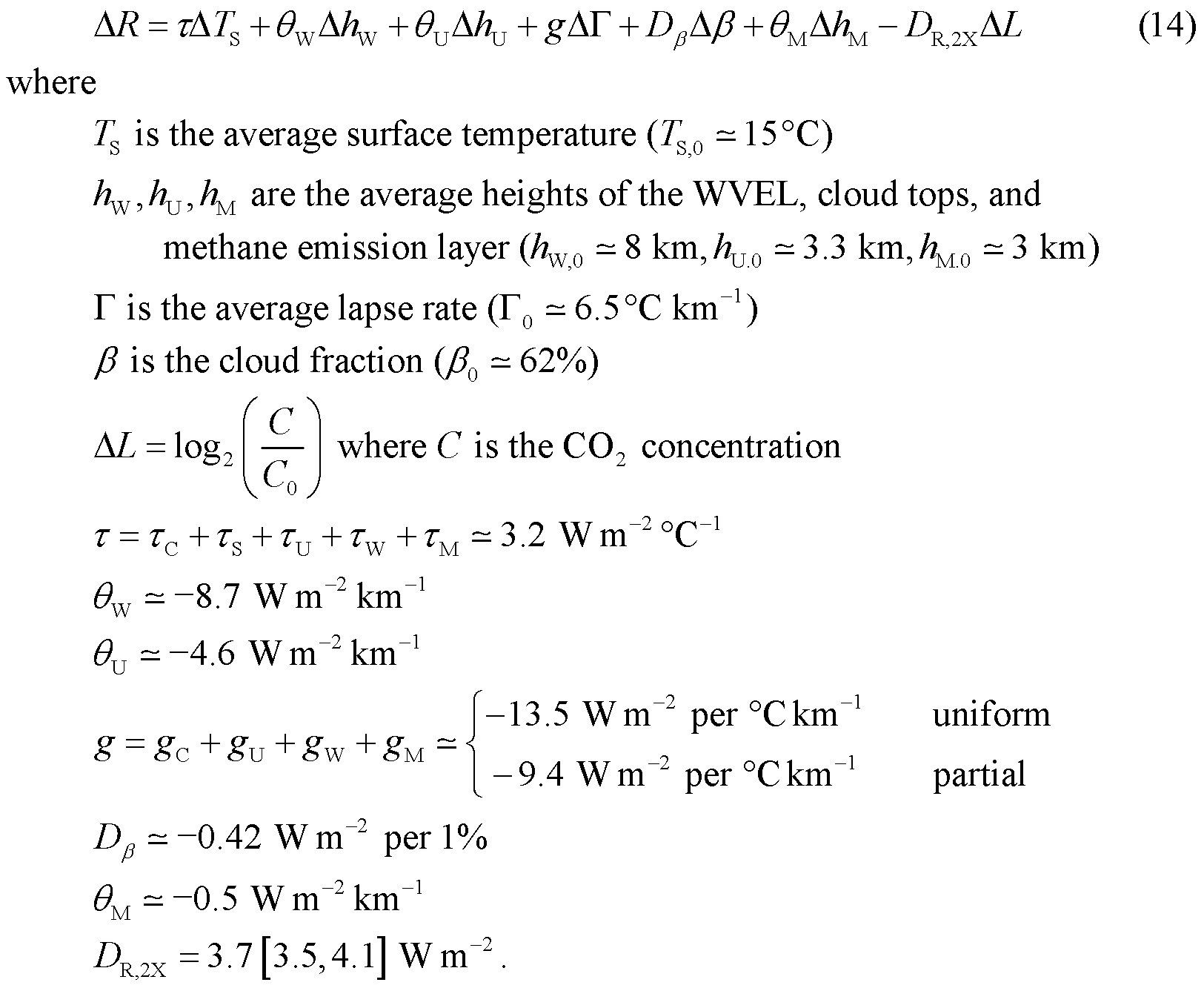### Diagram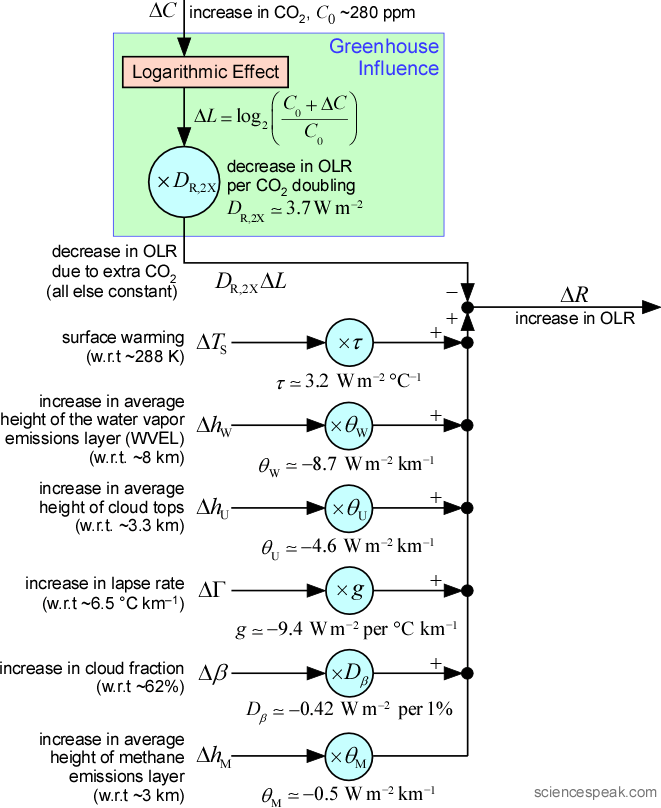Figure 2: The OLR model in Eq. (14), as a diagram. Beware: the seven inputs have complicated interdependencies. (Click to enlarge)

### Estimate of the Planck Sensitivity

In the OLR model of Eq. (14), τ is the Planck feedback, the reciprocal of the Planck sensitivity — it is the ratio of the increase in OLR to the increase in surface temperature, when everything else is held constant (the hypothetical Planck conditions — see post 2, post 4, and the Nomenclature file linked to above.). Its value is given in AR5 as 3.2±0.1 W m−2 °C−1, agreeing with the value here.

Over 80% of the Planck feedback’s value is due to the Stefan-Boltzmann law.  The Planck sensitivity λ0 (whose reciprocal is the Planck feedback) is 17% greater than λSB, the Stefan-Boltzmann sensitivity: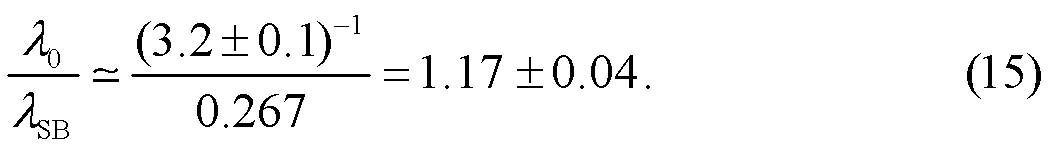Most of the rest of the difference between the Planck and Stefan-Boltzmann sensitivities is due to the non-Stefan-Boltzmann behavior of the CO2 and ozone emission layers — because they are mainly in the stratosphere, whose temperatures are constant under the Planck conditions, the amount of OLR they emit is less sensitive to surface warming than if they were totally in the troposphere. There is also a small effect due to the non-uniform distribution of surface temperature by time and latitude.

In the spreadsheet of the OLR model, by noting how the OLR changes when the surface temperature is incremented under the Planck conditions, the Planck sensitivity  λ0  is computed as 0.31 °C W−1 m2, or ~15.8% greater than λSB:

• 14.4% out of the 15.8% difference is due to the non-Stefan-Boltzmann behavior of the CO2 and ozone emission layers.
• The other 1.6% out of the 15.8% difference is due to the non-uniform temperature distribution and the concavity of the T4 function (0.36% due to temperature variation with latitude, 0.04% time of day, 0.03% seasons, and 0.97% due to the different temperatures of the main six emission layers).

That this estimates the Planck sensitivity as about the same as AR5 gives some support to the OLR model.

### References

[1^] Paltridge, G., Arking, A., & Pook, M. (2009). Trends in middle- and upper-level tropospheric humidity from NCEP reanalysis data. Theoretical and Applied Climatology, 98:351-359.

VN:F [1.9.22_1171]